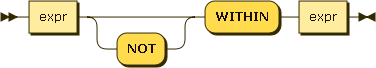# EVERY WITHIN != 'x' returns different results than NOT ANY WITHIN = 'x'

Given documents with this shape:

``````{
id: 12345,
{ q: 'question 1', v: 'answer1'},
]
},
{
id: 23456,
]
}
``````

I want to find all documents where none of the answers is for ‘question 1’. Question 1 may never be answered.

``````SELECT bucket.* FROM bucket WHERE type='document'
AND EVERY a WITHIN answers SATISFIES a.q <> 'question 1' END
``````

The query above returns 0 results (which is wrong), while the query below returns the correct number of results:

``````SELECT bucket.* FROM bucket WHERE type='document'
AND NOT ANY a WITHIN answers SATISFIES a.q = 'question 1'  END
``````

I don’t understand why the first and second queries aren’t functionally equivalent. What am I missing?

@Robert_Mirabelle This looks like you may have uncovered a bug. @vsr1 should be able to confirm.

WITHIN is recursive. EVERY a WITHIN answers SATISFIES a.q <> ‘question 1’ END will never be true.
EVERY is list of AND’s of each element every condition.

``````         Take id 23456
When a is answers it will be true,
next recursive a becomes q or v    q.q  or v.q is MISSING
Missing  SATISFIES will be false
So EVERY will be false
``````

You should use EVERY a IN answers SATISFIES a.q <> ‘question 1’ END

As mentioned EVERY LIST of ANDs every is not same as NOT ANY

@vsr1 Thanks for the info. I’m still confused at the difference between IN and WITHIN. If WITHIN is recursive and IN is not recursive, but the array of answers has no child/descendent arrays, why would there be any difference in the result?

Does this mean that when using `EVERY a WITHIN answers`, that what `a` currently represents is variable? First it represents an entire answer object (a = answers), then it changes to represent the first property of the current answer (a = q), then the second property of the current answer (a = v), and so on? Does `EVERY a WITHIN answers` really mean EVERY property WITHIN every answer?

Thanks again

Recursive means value of a becomes intermediate and all the way leaf each path including values.

Use ARRAY like below and see what it considers. If one want

`````` SELECT RAW ARRAY a FOR a  WITHIN { "id": 12345, "answers": [ { "q": 'question 1', "v": 'answer1'}, {"q": 'question 2',"v": 'answer2'} ] } END;
{
"signature": "array",
"results": [
[
[
{
"q": "question 1",
},
{
"q": "question 2",
}
],
{
"q": "question 1",
},
"question 1",
{
"q": "question 2",
},
"question 2",
12345
]
],
"status": "success",
"metrics": {
"elapsedTime": "1.213093ms",
"executionTime": "1.157678ms",
"resultCount": 1,
"resultSize": 471,
}
}
``````

#### ANY or SOME, EVERY, and ANY AND EVERY or SOME AND EVERY

Range predicates (ANY or SOME, EVERY, and ANY AND EVERY or SOME AND EVERY) allow you to test a boolean condition over the elements or attributes of a collection or object(s). They each evaluate to a boolean value.

ANY or SOME is TRUE if the collection is non-empty and at least one element matches.

EVERY is TRUE if the collection is empty, or if the collection is non-empty and every element matches.

ANY AND EVERY or SOME AND EVERY is TRUE if the collection is non-empty and every element matches.

#### WITHIN

within-expr:WITHIN evaluates to TRUE if the right-hand-side value contains the left-hand-side value (or name and value) as a child or descendant (i.e. directly or indirectly).